# A more accurate half-discrete Hilbert-type inequality with a non-homogeneous kernel

## Abstract

By means of weight functions and the improved Euler-Maclaurin summation formula, a more accurate half-discrete Hilbert-type inequality with a non-homogeneous kernel and a best constant factor is given. A best extension, some equivalent forms, the operator expressions as well as some particular cases are also considered.

MSC:26D15, 47A07.

## 1 Introduction

Assuming that $f,g\in {L}^{2}\left({\mathbf{R}}_{+}\right)$, $\parallel f\parallel ={\left\{{\int }_{0}^{\mathrm{\infty }}{f}^{2}\left(x\right)\phantom{\rule{0.2em}{0ex}}dx\right\}}^{\frac{1}{2}}>0$, $\parallel g\parallel >0$, we have the following Hilbert’s integral inequality (cf. ):

${\int }_{0}^{\mathrm{\infty }}{\int }_{0}^{\mathrm{\infty }}\frac{f\left(x\right)g\left(y\right)}{x+y}\phantom{\rule{0.2em}{0ex}}dx\phantom{\rule{0.2em}{0ex}}dy<\pi \parallel f\parallel \parallel g\parallel ,$
(1)

where the constant factor π is best possible. If $a={\left\{{a}_{n}\right\}}_{n=1}^{\mathrm{\infty }},b={\left\{{b}_{n}\right\}}_{n=1}^{\mathrm{\infty }}\in {l}^{2}$, $\parallel a\parallel ={\left\{{\sum }_{n=1}^{\mathrm{\infty }}{a}_{n}^{2}\right\}}^{\frac{1}{2}}>0$, $\parallel b\parallel >0$, then we have the following analogous discrete Hilbert’s inequality:

$\sum _{m=1}^{\mathrm{\infty }}\sum _{n=1}^{\mathrm{\infty }}\frac{{a}_{m}{b}_{n}}{m+n}<\pi \parallel a\parallel \parallel b\parallel ,$
(2)

with the same best constant factor π. Inequalities (1) and (2) are important in analysis and its applications (cf. ).

In 1998, by introducing an independent parameter $\lambda \in \left(0,1\right]$, Yang  gave an extension of (1). For generalizing the results from , Yang  gave some best extensions of (1) and (2) as follows. If $p>1$, $\frac{1}{p}+\frac{1}{q}=1$, ${\lambda }_{1}+{\lambda }_{2}=\lambda$, ${k}_{\lambda }\left(x,y\right)$ is a non-negative homogeneous function of degree −λ satisfying $k\left({\lambda }_{1}\right)={\int }_{0}^{\mathrm{\infty }}{k}_{\lambda }\left(t,1\right){t}^{{\lambda }_{1}-1}\phantom{\rule{0.2em}{0ex}}dt\in {\mathbf{R}}_{+}$, $\varphi \left(x\right)={x}^{p\left(1-{\lambda }_{1}\right)-1}$, $\psi \left(x\right)={x}^{q\left(1-{\lambda }_{2}\right)-1}$, $f\left(\ge 0\right)\in {L}_{p,\varphi }\left({\mathbf{R}}_{+}\right)=\left\{f|{\parallel f\parallel }_{p,\varphi }:={\left\{{\int }_{0}^{\mathrm{\infty }}\varphi \left(x\right){|f\left(x\right)|}^{p}\phantom{\rule{0.2em}{0ex}}dx\right\}}^{\frac{1}{p}}<\mathrm{\infty }\right\}$, $g\left(\ge 0\right)\in {L}_{q,\psi }\left({\mathbf{R}}_{+}\right)$, and ${\parallel f\parallel }_{p,\varphi }$, ${\parallel g\parallel }_{q,\psi }>0$, then

${\int }_{0}^{\mathrm{\infty }}{\int }_{0}^{\mathrm{\infty }}{k}_{\lambda }\left(x,y\right)f\left(x\right)g\left(y\right)\phantom{\rule{0.2em}{0ex}}dx\phantom{\rule{0.2em}{0ex}}dy
(3)

where the constant factor $k\left({\lambda }_{1}\right)$ is best possible. Moreover, if ${k}_{\lambda }\left(x,y\right)$ is finite and ${k}_{\lambda }\left(x,y\right){x}^{{\lambda }_{1}-1}\left({k}_{\lambda }\left(x,y\right){y}^{{\lambda }_{2}-1}\right)$ is decreasing for $x>0$ ($y>0$), then for ${a}_{m,}{b}_{n}\ge 0$, $a={\left\{{a}_{m}\right\}}_{m=1}^{\mathrm{\infty }}\in {l}_{p,\varphi }=\left\{a|{\parallel a\parallel }_{p,\varphi }:={\left\{{\sum }_{n=1}^{\mathrm{\infty }}\varphi \left(n\right){|{a}_{n}|}^{p}\right\}}^{\frac{1}{p}}<\mathrm{\infty }\right\}$, and $b={\left\{{b}_{n}\right\}}_{n=1}^{\mathrm{\infty }}\in {l}_{q,\psi }$, ${\parallel a\parallel }_{p,\varphi }$, ${\parallel b\parallel }_{q,\psi }>0$, we have

$\sum _{m=1}^{\mathrm{\infty }}\sum _{n=1}^{\mathrm{\infty }}{k}_{\lambda }\left(m,n\right){a}_{m}{b}_{n}
(4)

where the constant $k\left({\lambda }_{1}\right)$ is still the best value. Clearly, for $p=q=2$, $\lambda =1$, ${k}_{1}\left(x,y\right)=\frac{1}{x+y}$, ${\lambda }_{1}={\lambda }_{2}=\frac{1}{2}$, (3) reduces to (1), while (4) reduces to (2).

Some other results about integral and discrete Hilbert-type inequalities can be found in . On half-discrete Hilbert-type inequalities with the general non-homogeneous kernels, Hardy et al. provided a few results in Theorem 351 of . But they did not prove that the constant factors are best possible. In 2005, Yang  gave a result with the kernel $\frac{1}{{\left(1+nx\right)}^{\lambda }}$ by introducing a variable and proved that the constant factor is best possible. Very recently, Yang  and  gave the following half-discrete Hilbert’s inequality with the best constant factor:

${\int }_{0}^{\mathrm{\infty }}f\left(x\right)\sum _{n=1}^{\mathrm{\infty }}\frac{{a}_{n}}{{\left(x+n\right)}^{\lambda }}\phantom{\rule{0.2em}{0ex}}dx<\pi \parallel f\parallel \parallel a\parallel ;$
(5)

Chen  and Yang  gave two more accurate half-discrete Mulholland’s inequalities by using Hadamard’s inequality.

In this paper, by means of weight functions and the improved Euler-Maclaurin summation formula, a more accurate half-discrete Hilbert-type inequality with a non-homogeneous kernel and a best constant factor is given as follows. For $0<\alpha +\beta \le 2$, $\gamma \in \mathbf{R}$, $\eta \le 1-\frac{\alpha +\beta }{8}\left(1+\sqrt{3+\frac{4}{\alpha +\beta }}\right)$,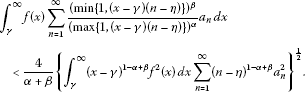(6)

Moreover, a best extension of (6), some equivalent forms, the operator expressions as well as some particular inequalities are considered.

## 2 Some lemmas

Lemma 1 If ${n}_{0}\in \mathbf{N}$, $s>{n}_{0}$, ${g}_{1}\left(y\right)$ ($y\in \left[{n}_{0},s\right)$), ${g}_{2}\left(y\right)$ ($y\in \left[s,\mathrm{\infty }\right)$) are decreasing continuous functions satisfying ${g}_{1}\left({n}_{0}\right)-{g}_{1}\left(s-0\right)+{g}_{2}\left(s\right)>0$, ${g}_{2}\left(\mathrm{\infty }\right)=0$, define a function $g\left(y\right)$ as follows:

$g\left(y\right):=\left\{\begin{array}{cc}{g}_{1}\left(y\right),\hfill & y\in \left[{n}_{0},s\right),\hfill \\ {g}_{2}\left(y\right),\hfill & y\in \left[s,\mathrm{\infty }\right).\hfill \end{array}$

Then there exists $\epsilon \in \left[0,1\right]$ such that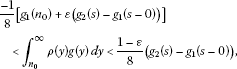(7)

where $\rho \left(y\right)=y-\left[y\right]-\frac{1}{2}$ is a Bernoulli function of the first order. In particular, for ${g}_{1}\left(y\right)=0$, $y\in \left[{n}_{0},s\right)$, we have ${g}_{2}\left(s\right)>0$ and

$\frac{-1}{8}{g}_{2}\left(s\right)<{\int }_{s}^{\mathrm{\infty }}\rho \left(y\right)g\left(y\right)\phantom{\rule{0.2em}{0ex}}dy<\frac{1}{8}{g}_{2}\left(s\right);$
(8)

for ${g}_{2}\left(y\right)=0$, $y\in \left[s,\mathrm{\infty }\right)$, if ${g}_{1}\left(s-0\right)\ge 0$, then it follows ${g}_{1}\left({n}_{0}\right)>0$ and

$\frac{-1}{8}{g}_{1}\left({n}_{0}\right)<{\int }_{{n}_{0}}^{s}\rho \left(y\right){g}_{1}\left(y\right)\phantom{\rule{0.2em}{0ex}}dy<0.$
(9)

Proof Define a decreasing continuous function $\stackrel{˜}{g}\left(y\right)$ as

$\stackrel{˜}{g}\left(y\right):=\left\{\begin{array}{cc}{g}_{1}\left(y\right)+{g}_{2}\left(s\right)-{g}_{1}\left(s-0\right),\hfill & y\in \left[{n}_{0},s\right),\hfill \\ {g}_{2}\left(y\right),\hfill & y\in \left[s,\mathrm{\infty }\right).\hfill \end{array}$

Then it follows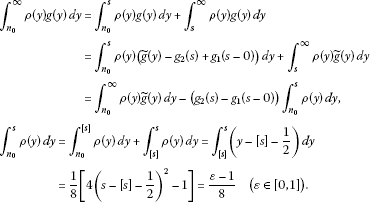Since $\stackrel{˜}{g}\left({n}_{0}\right)={g}_{1}\left({n}_{0}\right)+{g}_{2}\left(s\right)-{g}_{1}\left(s-0\right)>0$, $\stackrel{˜}{g}\left(y\right)$ is a non-constant decreasing continuous function with $\stackrel{˜}{g}\left(\mathrm{\infty }\right)={g}_{2}\left(\mathrm{\infty }\right)=0$, by the improved Euler-Maclaurin summation formula (cf. , Theorem 2.2.2), it follows

$\frac{-1}{8}\left({g}_{1}\left({n}_{0}\right)+{g}_{2}\left(s\right)-{g}_{1}\left(s-0\right)\right)=\frac{-1}{8}\stackrel{˜}{g}\left({n}_{0}\right)<{\int }_{{n}_{0}}^{\mathrm{\infty }}\rho \left(y\right)\stackrel{˜}{g}\left(y\right)\phantom{\rule{0.2em}{0ex}}dy<0,$

and then in view of the above results and by simple calculation, we have (7). □

Lemma 2 If $0<\alpha +\beta \le 2$, $\gamma \in \mathbf{R}$, $\eta \le 1-\frac{\alpha +\beta }{8}\left(1+\sqrt{3+\frac{4}{\alpha +\beta }}\right)$, and $\omega \left(n\right)$ and $\varpi \left(x\right)$ are weight functions given by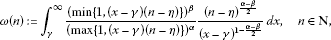(10)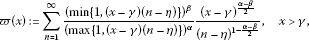(11)

then we have

$\varpi \left(x\right)<\omega \left(n\right)=\frac{4}{\alpha +\beta }.$
(12)

Proof Substituting $t=\left(x-\gamma \right)\left(n-\eta \right)$ in (10), and by simple calculation, we have

$\begin{array}{rcl}\omega \left(n\right)& =& {\int }_{0}^{\mathrm{\infty }}\frac{{\left(min\left\{1,t\right\}\right)}^{\beta }}{{\left(max\left\{1,t\right\}\right)}^{\alpha }}{t}^{\frac{\alpha -\beta }{2}-1}\phantom{\rule{0.2em}{0ex}}dt\\ =& {\int }_{0}^{1}{t}^{\beta +\frac{\alpha -\beta }{2}-1}\phantom{\rule{0.2em}{0ex}}dt+{\int }_{1}^{\mathrm{\infty }}{t}^{-\alpha +\frac{\alpha -\beta }{2}-1}\phantom{\rule{0.2em}{0ex}}dt=\frac{4}{\alpha +\beta }.\end{array}$

For fixed $x>\gamma$, we find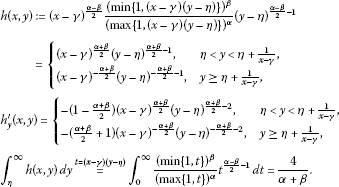By the Euler-Maclaurin summation formula (cf. ), it follows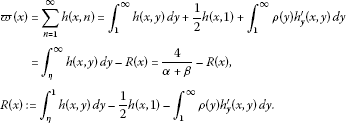(13)
1. (i)

For $0, we obtain $-\frac{1}{2}h\left(x,1\right)=-\frac{1}{2}{\left(x-\gamma \right)}^{\frac{\alpha +\beta }{2}}{\left(1-\eta \right)}^{\frac{\alpha +\beta }{2}-1}$, and

${\int }_{\eta }^{1}h\left(x,y\right)\phantom{\rule{0.2em}{0ex}}dy={\left(x-\gamma \right)}^{\frac{\alpha +\beta }{2}}{\int }_{\eta }^{1}{\left(y-\eta \right)}^{\frac{\alpha +\beta }{2}-1}\phantom{\rule{0.2em}{0ex}}dy=\frac{2{\left(1-\eta \right)}^{\frac{\alpha +\beta }{2}}}{\alpha +\beta }{\left(x-\gamma \right)}^{\frac{\alpha +\beta }{2}}.$

Setting $g\left(y\right):=-{h}_{y}^{\mathrm{\prime }}\left(x,y\right)$, wherefrom ${g}_{1}\left(y\right)=\left(1-\frac{\alpha +\beta }{2}\right){\left(x-\gamma \right)}^{\frac{\alpha +\beta }{2}}{\left(y-\eta \right)}^{\frac{\alpha +\beta }{2}-2}$, ${g}_{2}\left(y\right)=\left(\frac{\alpha +\beta }{2}+1\right){\left(x-\gamma \right)}^{-\frac{\alpha +\beta }{2}}{\left(y-\eta \right)}^{-\frac{\alpha +\beta }{2}-2}$ and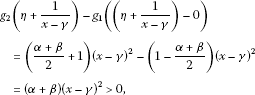then by (7), we find

$\begin{array}{rcl}-{\int }_{1}^{\mathrm{\infty }}\rho \left(y\right){h}_{y}^{\mathrm{\prime }}\left(x,y\right)\phantom{\rule{0.2em}{0ex}}dy& =& {\int }_{1}^{\mathrm{\infty }}\rho \left(y\right)g\left(y\right)\phantom{\rule{0.2em}{0ex}}dy\\ >& \frac{-1}{8}\left[{g}_{1}\left(1\right)+{g}_{2}\left(\eta +\frac{1}{x-\gamma }\right)-{g}_{1}\left(\left(\eta +\frac{1}{x-\gamma }\right)-0\right)\right]\\ =& \frac{-1}{8}\left[\left(1-\frac{\alpha +\beta }{2}\right){\left(x-\gamma \right)}^{\frac{\alpha +\beta }{2}}{\left(1-\eta \right)}^{\frac{\alpha +\beta }{2}-2}+\left(\alpha +\beta \right){\left(x-\gamma \right)}^{2}\right]\\ >& \frac{-1}{8}\left[\left(1-\frac{\alpha +\beta }{2}\right){\left(1-\eta \right)}^{\frac{\alpha +\beta }{2}-2}{\left(x-\gamma \right)}^{\frac{\alpha +\beta }{2}}\\ +\left(\alpha +\beta \right){\left(1-\eta \right)}^{\frac{\alpha +\beta }{2}-2}{\left(x-\gamma \right)}^{\frac{\alpha +\beta }{2}-2}{\left(x-\gamma \right)}^{2}\right]\\ =& \frac{-1}{8}\left[\left(1+\frac{\alpha +\beta }{2}\right){\left(1-\eta \right)}^{\frac{\alpha +\beta }{2}-2}{\left(x-\gamma \right)}^{\frac{\alpha +\beta }{2}}\right].\end{array}$

In view of (11) and the above results, since for $\eta \le 1-\frac{\alpha +\beta }{8}\left(1+\sqrt{3+\frac{4}{\alpha +\beta }}\right)$, namely $1-\eta \ge \frac{\alpha +\beta }{8}\left(1+\sqrt{3+\frac{4}{\alpha +\beta }}\right)$, it follows

$\begin{array}{rcl}R\left(x\right)& >& \frac{2}{\alpha +\beta }{\left(1-\eta \right)}^{\frac{\alpha +\beta }{2}}{\left(x-\gamma \right)}^{\frac{\alpha +\beta }{2}}-\frac{1}{2}{\left(x-\gamma \right)}^{\frac{\alpha +\beta }{2}}{\left(1-\eta \right)}^{\frac{\alpha +\beta }{2}-1}\\ -\frac{1}{8}\left(1+\frac{\alpha +\beta }{2}\right){\left(1-\eta \right)}^{\frac{\alpha +\beta }{2}-2}{\left(x-\gamma \right)}^{\frac{\alpha +\beta }{2}}\\ =& \left[\frac{2{\left(1-\eta \right)}^{2}}{\alpha +\beta }-\frac{\left(1-\eta \right)}{2}-\frac{2+\alpha +\beta }{16}\right]\frac{{\left(x-\gamma \right)}^{\frac{\alpha +\beta }{2}}}{{\left(1-\eta \right)}^{2-\frac{\alpha +\beta }{2}}}\ge 0.\end{array}$
1. (ii)

For $x-\gamma \ge \frac{1}{1-\eta }$, we obtain $-\frac{1}{2}h\left(x,1\right)=-\frac{1}{2}{\left(x-\gamma \right)}^{-\frac{\alpha +\beta }{2}}{\left(1-\eta \right)}^{-\frac{\alpha +\beta }{2}-1}$, and

$\begin{array}{rcl}{\int }_{\eta }^{1}h\left(x,y\right)\phantom{\rule{0.2em}{0ex}}dy& =& {\int }_{\eta }^{\eta +\frac{1}{x-\gamma }}\frac{{\left(x-\gamma \right)}^{\frac{\alpha +\beta }{2}}}{{\left(y-\eta \right)}^{1-\frac{\alpha +\beta }{2}}}\phantom{\rule{0.2em}{0ex}}dy+{\int }_{\eta +\frac{1}{x-\gamma }}^{1}\frac{{\left(x-\gamma \right)}^{-\frac{\alpha +\beta }{2}}}{{\left(y-\eta \right)}^{\frac{\alpha +\beta }{2}+1}}\phantom{\rule{0.2em}{0ex}}dy\\ =& \frac{4}{\alpha +\beta }-\frac{2}{\alpha +\beta }{\left(1-\eta \right)}^{-\frac{\alpha +\beta }{2}}{\left(x-\gamma \right)}^{-\frac{\alpha +\beta }{2}}\\ \ge & \frac{4{\left(1-\eta \right)}^{-\frac{\alpha +\beta }{2}}}{\alpha +\beta }{\left(x-\gamma \right)}^{-\frac{\alpha +\beta }{2}}-\frac{2{\left(1-\eta \right)}^{-\frac{\alpha +\beta }{2}}}{\alpha +\beta }{\left(x-\gamma \right)}^{-\frac{\alpha +\beta }{2}}\\ =& \frac{2}{\alpha +\beta }{\left(1-\eta \right)}^{-\frac{\alpha +\beta }{2}}{\left(x-\gamma \right)}^{-\frac{\alpha +\beta }{2}}.\end{array}$

Since for $y\ge 1$, $y-\eta \ge \frac{1}{x-\gamma }$, by the improved Euler-Maclaurin summation formula (cf. ), it follows

$\begin{array}{rcl}-{\int }_{1}^{\mathrm{\infty }}\rho \left(y\right){h}_{y}^{\mathrm{\prime }}\left(x,y\right)\phantom{\rule{0.2em}{0ex}}dy& =& \left(\frac{\alpha +\beta }{2}+1\right){\left(x-\gamma \right)}^{-\frac{\alpha +\beta }{2}}{\int }_{1}^{\mathrm{\infty }}\rho \left(y\right){\left(y-\eta \right)}^{-\frac{\alpha +\beta }{2}-2}\phantom{\rule{0.2em}{0ex}}dy\\ >& -\frac{1}{12}\left(\frac{\alpha +\beta }{2}+1\right){\left(x-\gamma \right)}^{-\frac{\alpha +\beta }{2}}{\left(1-\eta \right)}^{-\frac{\alpha +\beta }{2}-2}.\end{array}$

In view of (13) and the above results, for $1-\eta \ge \frac{\alpha +\beta }{8}\left(1+\sqrt{3+\frac{4}{\alpha +\beta }}\right)$, we find

$\begin{array}{rcl}R\left(x\right)& >& \frac{2}{\alpha +\beta }{\left(1-\eta \right)}^{-\frac{\alpha +\beta }{2}}{\left(x-\gamma \right)}^{-\frac{\alpha +\beta }{2}}-\frac{1}{2}{\left(1-\eta \right)}^{-\frac{\alpha +\beta }{2}-1}{\left(x-\gamma \right)}^{-\frac{\alpha +\beta }{2}}\\ -\frac{1}{12}\left(\frac{\alpha +\beta }{2}+1\right){\left(1-\eta \right)}^{-\frac{\alpha +\beta }{2}-2}{\left(x-\gamma \right)}^{-\frac{\alpha +\beta }{2}}\\ >& \left[\frac{2{\left(1-\eta \right)}^{2}}{\alpha +\beta }-\frac{1-\eta }{2}-\frac{2+\alpha +\beta }{16}\right]\frac{{\left(x-\gamma \right)}^{-\frac{\alpha +\beta }{2}}}{{\left(1-\eta \right)}^{2+\frac{\alpha +\beta }{2}}}\ge 0.\end{array}$

Hence, for $x>\gamma$, we have $R\left(x\right)>0$, and then (12) follows. □

Lemma 3 Let the assumptions of Lemma 2 be fulfilled and, additionally, let $p>1$, $\frac{1}{p}+\frac{1}{q}=1$, ${a}_{n}\ge 0$, $n\in \mathbf{N}$, $f\left(x\right)$ be a non-negative measurable function in $\left(\gamma ,\mathrm{\infty }\right)$. Then we have the following inequalities: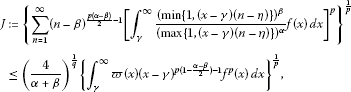(14)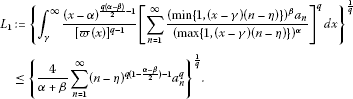(15)

Proof Setting $k\left(x,n\right):=\frac{{\left(min\left\{1,\left(x-\gamma \right)\left(n-\eta \right)\right\}\right)}^{\beta }}{{\left(max\left\{1,\left(x-\gamma \right)\left(n-\eta \right)\right\}\right)}^{\alpha }}$, by Hölder’s inequality (cf. ) and (12), it followsThen by the Lebesgue term-by-term integration theorem (cf. ), we have

$\begin{array}{rcl}J& \le & {\left(\frac{4}{\alpha +\beta }\right)}^{\frac{1}{q}}{\left\{\sum _{n=1}^{\mathrm{\infty }}{\int }_{\gamma }^{\mathrm{\infty }}k\left(x,n\right)\frac{{\left(x-\gamma \right)}^{\left(1-\frac{\alpha -\beta }{2}\right)\left(p-1\right)}}{{\left(n-\eta \right)}^{1-\frac{\alpha -\beta }{2}}}{f}^{p}\left(x\right)\phantom{\rule{0.2em}{0ex}}dx\right\}}^{\frac{1}{p}}\\ =& {\left(\frac{4}{\alpha +\beta }\right)}^{\frac{1}{q}}{\left\{{\int }_{\gamma }^{\mathrm{\infty }}\sum _{n=1}^{\mathrm{\infty }}k\left(x,n\right)\frac{{\left(x-\gamma \right)}^{\left(1-\frac{\alpha -\beta }{2}\right)\left(p-1\right)}}{{\left(n-\eta \right)}^{1-\frac{\alpha -\beta }{2}}}{f}^{p}\left(x\right)\phantom{\rule{0.2em}{0ex}}dx\right\}}^{\frac{1}{p}}\\ =& {\left(\frac{4}{\alpha +\beta }\right)}^{\frac{1}{q}}{\left\{{\int }_{\gamma }^{\mathrm{\infty }}\varpi \left(x\right){\left(x-\gamma \right)}^{p\left(1-\frac{\alpha -\beta }{2}\right)-1}{f}^{p}\left(x\right)\phantom{\rule{0.2em}{0ex}}dx\right\}}^{\frac{1}{p}}.\end{array}$

Hence, (14) follows. By Hölder’s inequality again, we have

$\begin{array}{rcl}{\left[\sum _{n=1}^{\mathrm{\infty }}k\left(x,n\right){a}_{n}\right]}^{q}& =& \left\{\sum _{n=1}^{\mathrm{\infty }}k\left(x,n\right)\left[\frac{{\left(x-\gamma \right)}^{\left(1-\frac{\alpha -\beta }{2}\right)/q}}{{\left(n-\eta \right)}^{\left(1-\frac{\alpha -\beta }{2}\right)/p}}\right]\\ ×\left[\frac{{\left(n-\eta \right)}^{\left(1-\frac{\alpha -\beta }{2}\right)/p}{a}_{n}}{{\left(x-\gamma \right)}^{\left(1-\frac{\alpha -\beta }{2}\right)/q}}\right]{\right\}}^{q}\le {\left\{\sum _{n=1}^{\mathrm{\infty }}k\left(x,n\right)\frac{{\left(x-\gamma \right)}^{\left(1-\frac{\alpha -\beta }{2}\right)\left(p-1\right)}}{{\left(n-\eta \right)}^{1-\frac{\alpha -\beta }{2}}}\right\}}^{q-1}\\ ×\sum _{n=1}^{\mathrm{\infty }}k\left(x,n\right)\frac{{\left(n-\eta \right)}^{\left(1-\frac{\alpha -\beta }{2}\right)\left(q-1\right)}}{{\left(x-\gamma \right)}^{1-\frac{\alpha -\beta }{2}}}{a}_{n}^{q}\\ =& \frac{{\left[\varpi \left(x\right)\right]}^{q-1}}{{\left(x-\gamma \right)}^{\frac{q\left(\alpha -\beta \right)}{2}-1}}\sum _{n=1}^{\mathrm{\infty }}k\left(x,n\right)\frac{{\left(n-\eta \right)}^{\left(1-\frac{\alpha -\beta }{2}\right)\left(q-1\right)}}{{\left(x-\gamma \right)}^{1-\frac{\alpha -\beta }{2}}}{a}_{n}^{q}.\end{array}$

By the Lebesgue term-by-term integration theorem, we have

$\begin{array}{rcl}{L}_{1}& \le & {\left\{{\int }_{\gamma }^{\mathrm{\infty }}\sum _{n=1}^{\mathrm{\infty }}k\left(x,n\right)\frac{{\left(n-\eta \right)}^{\left(1-\frac{\alpha -\beta }{2}\right)\left(q-1\right)}}{{\left(x-\gamma \right)}^{1-\frac{\alpha -\beta }{2}}}{a}_{n}^{q}\phantom{\rule{0.2em}{0ex}}dx\right\}}^{\frac{1}{q}}\\ =& {\left\{\sum _{n=1}^{\mathrm{\infty }}{\int }_{\gamma }^{\mathrm{\infty }}k\left(x,n\right)\frac{{\left(n-\eta \right)}^{\left(1-\frac{\alpha -\beta }{2}\right)\left(q-1\right)}}{{\left(x-\gamma \right)}^{1-\frac{\alpha -\beta }{2}}}{a}_{n}^{q}\phantom{\rule{0.2em}{0ex}}dx\right\}}^{\frac{1}{q}}\\ =& {\left\{\sum _{n=1}^{\mathrm{\infty }}\omega \left(n\right){\left(n-\eta \right)}^{q\left(1-\frac{\alpha -\beta }{2}\right)-1}{a}_{n}^{q}\right\}}^{\frac{1}{q}},\end{array}$

and in view of (12), inequality (15) follows. □

Lemma 4 Let the assumptions of Lemma 2 be fulfilled and, additionally, let $p>1$, $\frac{1}{p}+\frac{1}{q}=1$, $0<\epsilon <\frac{p}{2}\left(\alpha +\beta \right)$. Setting $\stackrel{˜}{f}\left(x\right)={\left(x-\gamma \right)}^{\frac{\alpha -\beta }{2}+\frac{\epsilon }{p}-1}$, $x\in \left(\gamma ,\gamma +1\right)$; $\stackrel{˜}{f}\left(x\right)=0$, $x\in \left[\gamma +1,\mathrm{\infty }\right)$, and ${\stackrel{˜}{a}}_{n}={\left(n-\eta \right)}^{\frac{\alpha -\beta }{2}-\frac{\epsilon }{q}-1}$, $n\in \mathbf{N}$, then we have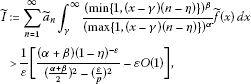(16)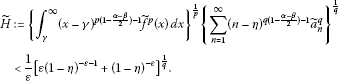(17)

Proof We find

$\begin{array}{rcl}\stackrel{˜}{I}& =& \sum _{n=1}^{\mathrm{\infty }}{\left(n-\eta \right)}^{\frac{\alpha -\beta }{2}-\frac{\epsilon }{q}-1}\\ ×{\int }_{\gamma }^{\gamma +1}\frac{{\left(min\left\{1,\left(x-\gamma \right)\left(n-\eta \right)\right\}\right)}^{\beta }}{{\left(max\left\{1,\left(x-\gamma \right)\left(n-\eta \right)\right\}\right)}^{\alpha }}{\left(x-\gamma \right)}^{\frac{\alpha -\beta }{2}+\frac{\epsilon }{p}-1}\phantom{\rule{0.2em}{0ex}}dx\\ =& \sum _{n=1}^{\mathrm{\infty }}{\left(n-\eta \right)}^{\frac{\alpha -\beta }{2}-\frac{\epsilon }{q}-1}\left[{\left(n-\eta \right)}^{\beta }{\int }_{\gamma }^{\gamma +\frac{1}{n-\eta }}{\left(x-\gamma \right)}^{\frac{\alpha +\beta }{2}+\frac{\epsilon }{p}-1}\phantom{\rule{0.2em}{0ex}}dx\\ +\frac{1}{{\left(n-\eta \right)}^{\alpha }}{\int }_{\gamma +\frac{1}{n-\eta }}^{\gamma +1}{\left(x-\gamma \right)}^{-\frac{\alpha +\beta }{2}+\frac{\epsilon }{p}-1}\phantom{\rule{0.2em}{0ex}}dx\right]\\ =& \frac{\alpha +\beta }{{\left(\frac{\alpha +\beta }{2}\right)}^{2}-{\left(\frac{\epsilon }{p}\right)}^{2}}\sum _{n=1}^{\mathrm{\infty }}{\left(n-\eta \right)}^{-\epsilon -1}-\frac{1}{\frac{\alpha +\beta }{2}-\frac{\epsilon }{p}}\sum _{n=1}^{\mathrm{\infty }}{\left(n-\eta \right)}^{-\frac{\alpha +\beta }{2}-\frac{\epsilon }{q}-1}\\ >& \frac{\alpha +\beta }{{\left(\frac{\alpha +\beta }{2}\right)}^{2}-{\left(\frac{\epsilon }{p}\right)}^{2}}{\int }_{1}^{\mathrm{\infty }}\frac{dy}{{\left(y-\eta \right)}^{\epsilon +1}}-\frac{1}{\frac{\alpha +\beta }{2}-\frac{\epsilon }{p}}\sum _{n=1}^{\mathrm{\infty }}{\left(n-\eta \right)}^{-\frac{\alpha +\beta }{2}-\frac{\epsilon }{q}-1}\\ =& \frac{1}{\epsilon }\left[\frac{\left(\alpha +\beta \right){\left(1-\eta \right)}^{-\epsilon }}{{\left(\frac{\alpha +\beta }{2}\right)}^{2}-{\left(\frac{\epsilon }{p}\right)}^{2}}-\frac{\epsilon }{\frac{\alpha +\beta }{2}-\frac{\epsilon }{p}}\sum _{n=1}^{\mathrm{\infty }}{\left(n-\eta \right)}^{-\frac{\alpha +\beta }{2}-\frac{\epsilon }{q}-1}\right],\end{array}$

and then (16) is valid. We obtain

$\begin{array}{rcl}\stackrel{˜}{H}& =& {\left\{{\int }_{\gamma }^{\gamma +1}{\left(x-\gamma \right)}^{\epsilon -1}\phantom{\rule{0.2em}{0ex}}dx\right\}}^{\frac{1}{p}}{\left\{{\left(1-\eta \right)}^{-\epsilon -1}+\sum _{n=2}^{\mathrm{\infty }}{\left(n-\eta \right)}^{-\epsilon -1}\right\}}^{\frac{1}{q}}\\ <& {\left(\frac{1}{\epsilon }\right)}^{\frac{1}{p}}{\left\{{\left(1-\eta \right)}^{-\epsilon -1}+{\int }_{1}^{\mathrm{\infty }}{\left(y-\eta \right)}^{-\epsilon -1}\phantom{\rule{0.2em}{0ex}}dy\right\}}^{\frac{1}{q}}\\ =& \frac{1}{\epsilon }{\left\{\epsilon {\left(1-\eta \right)}^{-\epsilon -1}+{\left(1-\eta \right)}^{-\epsilon }\right\}}^{\frac{1}{q}},\end{array}$

and so (17) is valid. □

## 3 Main results

We introduce the functions

$\mathrm{\Phi }\left(x\right):={\left(x-\gamma \right)}^{p\left(1-\frac{\alpha -\beta }{2}\right)-1}\phantom{\rule{1em}{0ex}}\left(x\in \left(\gamma ,\mathrm{\infty }\right)\right),\phantom{\rule{2em}{0ex}}\mathrm{\Psi }\left(n\right):={\left(n-\eta \right)}^{q\left(1-\frac{\alpha -\beta }{2}\right)-1}\phantom{\rule{1em}{0ex}}\left(n\in \mathbf{N}\right),$

wherefrom ${\left[\mathrm{\Phi }\left(x\right)\right]}^{1-q}={\left(x-\gamma \right)}^{q\frac{\alpha -\beta }{2}-1}$ and ${\left[\mathrm{\Psi }\left(n\right)\right]}^{1-p}={\left(n-\eta \right)}^{p\frac{\alpha -\beta }{2}-1}$.

Theorem 5 If $0<\alpha +\beta \le 2$, $\gamma \in \mathbf{R}$, $\eta \le 1-\frac{\alpha +\beta }{8}\left(1+\sqrt{3+\frac{4}{\alpha +\beta }}\right)$, $p>1$, $\frac{1}{p}+\frac{1}{q}=1$, $f\left(x\right),{a}_{n}\ge 0$, $f\in {L}_{p,\mathrm{\Phi }}\left(\gamma ,\mathrm{\infty }\right)$, $a={\left\{{a}_{n}\right\}}_{n=1}^{\mathrm{\infty }}\in {l}_{q,\mathrm{\Psi }}$, ${\parallel f\parallel }_{p,\mathrm{\Phi }}>0$ and ${\parallel a\parallel }_{q,\mathrm{\Psi }}>0$, then we have the following equivalent inequalities: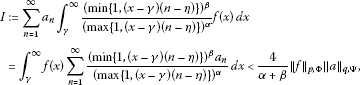(18)(19)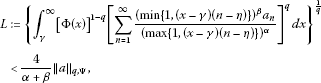(20)

where the constant $\frac{4}{\alpha +\beta }$ is the best possible in the above inequalities.

Proof The two expressions for I in (18) follow from the Lebesgue term-by-term integration theorem. By (14) and (12), we have (19). By Hölder’s inequality, we have

$\begin{array}{rcl}I& =& \sum _{n=1}^{\mathrm{\infty }}\left[{\mathrm{\Psi }}^{\frac{-1}{q}}\left(n\right){\int }_{\gamma }^{\mathrm{\infty }}\frac{{\left(min\left\{1,\left(x-\gamma \right)\left(n-\eta \right)\right\}\right)}^{\beta }f\left(x\right)}{{\left(max\left\{1,\left(x-\gamma \right)\left(n-\eta \right)\right\}\right)}^{\alpha }}\phantom{\rule{0.2em}{0ex}}dx\right]\left[{\mathrm{\Psi }}^{\frac{1}{q}}\left(n\right){a}_{n}\right]\\ \le & J{\parallel a\parallel }_{q,\mathrm{\Psi }}.\end{array}$

Then by (19), we have (18). On the other hand, assume that (18) is valid. Setting

${a}_{n}:={\left[\mathrm{\Psi }\left(n\right)\right]}^{1-p}{\left[{\int }_{\gamma }^{\mathrm{\infty }}\frac{{\left(min\left\{1,\left(x-\gamma \right)\left(n-\eta \right)\right\}\right)}^{\beta }f\left(x\right)}{{\left(max\left\{1,\left(x-\gamma \right)\left(n-\eta \right)\right\}\right)}^{\alpha }}\phantom{\rule{0.2em}{0ex}}dx\right]}^{p-1},\phantom{\rule{1em}{0ex}}n\in \mathbf{N},$

where ${J}^{p-1}={\parallel a\parallel }_{q,\mathrm{\Psi }}$. By (14), we find $J<\mathrm{\infty }$. If $J=0$, then (19) is trivially valid; if $J>0$, then by (18) we have

${\parallel a\parallel }_{q,\mathrm{\Psi }}^{q}={J}^{q\left(p-1\right)}={J}^{p}=I<\frac{4}{\alpha +\beta }{\parallel f\parallel }_{p,\mathrm{\Phi }}{\parallel a\parallel }_{q,\mathrm{\Psi }},$

therefore ${\parallel a\parallel }_{q,\mathrm{\Psi }}^{q-1}=J<\frac{4}{\alpha +\beta }{\parallel f\parallel }_{p,\mathrm{\Phi }}$; that is, (19) is equivalent to (18). On the other hand, by (12) we have ${\left[\varpi \left(x\right)\right]}^{1-q}>{\left(\frac{4}{\alpha +\beta }\right)}^{1-q}$. Then in view of (15), we have (20). By Hölder’s inequality, we find

$\begin{array}{rcl}I& =& {\int }_{\gamma }^{\mathrm{\infty }}\left[{\mathrm{\Phi }}^{\frac{1}{p}}\left(x\right)f\left(x\right)\right]\left[{\mathrm{\Phi }}^{\frac{-1}{p}}\left(x\right)\sum _{n=1}^{\mathrm{\infty }}\frac{{\left(min\left\{1,\left(x-\gamma \right)\left(n-\eta \right)\right\}\right)}^{\beta }{a}_{n}}{{\left(max\left\{1,\left(x-\gamma \right)\left(n-\eta \right)\right\}\right)}^{\alpha }}\right]\phantom{\rule{0.2em}{0ex}}dx\\ \le & {\parallel f\parallel }_{p,\mathrm{\Phi }}L.\end{array}$

Then by (20), we have (18). On the other hand, assume that (18) is valid. Setting

$f\left(x\right):={\left[\mathrm{\Phi }\left(x\right)\right]}^{1-q}{\left[\sum _{n=1}^{\mathrm{\infty }}\frac{{\left(min\left\{1,\left(x-\gamma \right)\left(n-\eta \right)\right\}\right)}^{\beta }{a}_{n}}{{\left(max\left\{1,\left(x-\gamma \right)\left(n-\eta \right)\right\}\right)}^{\alpha }}\right]}^{q-1},\phantom{\rule{1em}{0ex}}x\in \left(\gamma ,\mathrm{\infty }\right),$

then ${L}^{q-1}={\parallel f\parallel }_{p,\mathrm{\Phi }}$. By (15), we find $L<\mathrm{\infty }$. If $L=0$, then (20) is trivially valid; if $L>0$, then by (18), we have

${\parallel f\parallel }_{p,\mathrm{\Phi }}^{p}={L}^{p\left(q-1\right)}=I<\frac{4}{\alpha +\beta }{\parallel f\parallel }_{p,\mathrm{\Phi }}{\parallel a\parallel }_{q,\mathrm{\Psi }},$

therefore ${\parallel f\parallel }_{p,\mathrm{\Phi }}^{p-1}=L<\frac{4}{\alpha +\beta }{\parallel a\parallel }_{q,\mathrm{\Psi }}$; that is, (20) is equivalent to (18). Hence, (18), (19) and (20) are equivalent.

If there exists a positive number k ($\le \frac{4}{\alpha +\beta }$) such that (18) is valid as we replace $\frac{4}{\alpha +\beta }$ with k, then, in particular, it follows that $\stackrel{˜}{I}. In view of (16) and (17), we have

$\frac{\left(\alpha +\beta \right){\left(1-\eta \right)}^{-\epsilon }}{{\left(\frac{\alpha +\beta }{2}\right)}^{2}-{\left(\frac{\epsilon }{p}\right)}^{2}}-\epsilon O\left(1\right)

and $\frac{4}{\alpha +\beta }\le k$ ($\epsilon \to {0}^{+}$). Hence, $k=\frac{4}{\alpha +\beta }$ is the best value of (18).

By the equivalence of the inequalities, the constant factor $\frac{4}{\alpha +\beta }$ in (19) and (20) is the best possible. □

Remark 1 (i) Define the first type half-discrete Hilbert-type operator ${T}_{1}:{L}_{p,\mathrm{\Phi }}\left(\gamma ,\mathrm{\infty }\right)\to {l}_{p,{\mathrm{\Psi }}^{1-p}}$ as follows. For $f\in {L}_{p,\mathrm{\Phi }}\left(\gamma ,\mathrm{\infty }\right)$, we define ${T}_{1}f\in {l}_{p,{\mathrm{\Psi }}^{1-p}}$ by

${T}_{1}f\left(n\right)={\int }_{\gamma }^{\mathrm{\infty }}\frac{{\left(min\left\{1,\left(x-\gamma \right)\left(n-\eta \right)\right\}\right)}^{\beta }}{{\left(max\left\{1,\left(x-\gamma \right)\left(n-\eta \right)\right\}\right)}^{\alpha }}f\left(x\right)\phantom{\rule{0.2em}{0ex}}dx,\phantom{\rule{1em}{0ex}}n\in \mathbf{N}.$

Then by (19), ${\parallel {T}_{1}f\parallel }_{p,{\mathrm{\Psi }}^{1-p}}\le \frac{4}{\alpha +\beta }{\parallel f\parallel }_{p,\mathrm{\Phi }}$ and so ${T}_{1}$ is a bounded operator with $\parallel {T}_{1}\parallel \le \frac{4}{\alpha +\beta }$. Since by Theorem 5 the constant factor in (19) is best possible, we have $\parallel {T}_{1}\parallel =\frac{4}{\alpha +\beta }$.

1. (ii)

Define the second type half-discrete Hilbert-type operator ${T}_{2}:{l}_{q,\mathrm{\Psi }}\to {L}_{q,{\mathrm{\Phi }}^{1-q}}\left(\gamma ,\mathrm{\infty }\right)$ as follows. For $a\in {l}_{q,\mathrm{\Psi }}$, we define ${T}_{2}a\in {L}_{q,{\mathrm{\Phi }}^{1-q}}\left(\gamma ,\mathrm{\infty }\right)$ by

${T}_{2}a\left(x\right)=\sum _{n=1}^{\mathrm{\infty }}\frac{{\left(min\left\{1,\left(x-\gamma \right)\left(n-\eta \right)\right\}\right)}^{\beta }}{{\left(max\left\{1,\left(x-\gamma \right)\left(n-\eta \right)\right\}\right)}^{\alpha }}{a}_{n},\phantom{\rule{1em}{0ex}}x\in \left(\gamma ,\mathrm{\infty }\right).$

Then by (20), ${\parallel {T}_{2}a\parallel }_{q,{\mathrm{\Phi }}^{1-q}}\le \frac{4}{\alpha +\beta }{\parallel a\parallel }_{q,\mathrm{\Psi }}$ and so ${T}_{2}$ is a bounded operator with $\parallel {T}_{2}\parallel \le \frac{4}{\alpha +\beta }$. Since by Theorem 5 the constant factor in (20) is best possible, we have $\parallel {T}_{2}\parallel =\frac{4}{\alpha +\beta }$.

Remark 2 (i) For $p=q=2$, (18) reduces to (6). Since we find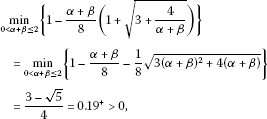then for $\eta =\gamma =0$ in (18), we have the following inequality: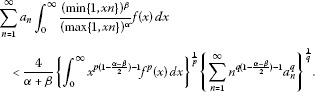(21)

Hence, (18) is a more accurate inequality of (21).

1. (ii)

For $\beta =0$ in (18), we have $0<\alpha \le 2$, $\gamma \in \mathbf{R}$, $\eta \le 1-\frac{\alpha }{8}\left(1+\sqrt{3+\frac{4}{\alpha }}\right)$, and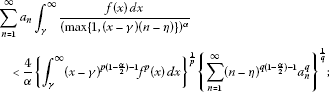(22)

for $\alpha =0$ in (18), we have $0<\beta \le 2$, $\gamma \in \mathbf{R}$, $\eta \le 1-\frac{\beta }{8}\left(1+\sqrt{3+\frac{4}{\beta }}\right)$, and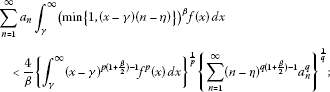(23)

for $\beta =\alpha =\lambda$ in (18), we have $0<\lambda \le 1$, $\gamma \in \mathbf{R}$, $\eta \le 1-\frac{\lambda }{4}\left(1+\sqrt{3+\frac{2}{\lambda }}\right)$, and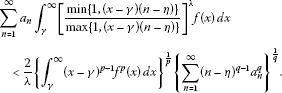(24)

## References

1. Hardy GH, Littlewood JE, Pólya G: Inequalities. Cambridge University Press, Cambridge; 1934.

2. Mitrinović DS, Pečarić JE, Fink AM: Inequalities Involving Functions and Their Integrals and Derivatives. Kluwer Academic, Boston; 1991.

3. Yang B: Hilbert-Type Integral Inequalities. Bentham Science Publishers, Sharjah; 2009.

4. Yang B: Discrete Hilbert-Type Inequalities. Bentham Science Publishers, Sharjah; 2011.

5. Yang B: On Hilbert’s integral inequality. J. Math. Anal. Appl. 1998, 220: 778–785. 10.1006/jmaa.1997.5877

6. Yang B: The Norm of Operator and Hilbert-Type Inequalities. Science Press, Beijing; 2009.

7. Yang B, Brnetić I, Krnić M, Pečarić J: Generalization of Hilbert and Hardy-Hilbert integral inequalities. Math. Inequal. Appl. 2005, 8(2):259–272.

8. Krnić M, Pečarić J: Hilbert’s inequalities and their reverses. Publ. Math. (Debr.) 2005, 67(3–4):315–331.

9. Jin J, Debnath L: On a Hilbert-type linear series operator and its applications. J. Math. Anal. Appl. 2010, 371: 691–704. 10.1016/j.jmaa.2010.06.002

10. Azar L: On some extensions of Hardy-Hilbert’s inequality and applications. J. Inequal. Appl. 2008., 2008: Article ID 546829

11. Yang B, Rassias T: On the way of weight coefficient and research for Hilbert-type inequalities. Math. Inequal. Appl. 2003, 6(4):625–658.

12. Arpad B, Choonghong O: Best constant for certain multilinear integral operator. J. Inequal. Appl. 2006., 2006: Article ID 28582

13. Kuang J, Debnath L: On Hilbert’s type inequalities on the weighted Orlicz spaces, pacific. J. Appl. Math. 2007, 1(1):95–103.

14. Zhong W: The Hilbert-type integral inequality with a homogeneous kernel of λ -degree. J. Inequal. Appl. 2008., 2008: Article ID 917392

15. Yang B: A new Hilbert-type operator and applications. Publ. Math. (Debr.) 2010, 76(1–2):147–156.

16. Li Y, He B: On inequalities of Hilbert’s type. Bull. Aust. Math. Soc. 2007, 76(1):1–13. 10.1017/S0004972700039423

17. Yang B: A mixed Hilbert-type inequality with a best constant factor. Int. J. Pure Appl. Math. 2005, 20(3):319–328.

18. Yang B: A half-discrete Hilbert’s inequality. J. Guangdong Educ. Inst. 2011, 31(3):1–7.

19. Yang B, Chen Q: A half-discrete Hilbert-type inequality with a homogeneous kernel and an extension. J. Inequal. Appl. 2011., 2011: Article ID 124. doi:10.1186/1029–242X-2011–124

20. Chen Q, Yang B: On a more accurate half-discrete Mulholland’s inequality and an extension. J. Inequal. Appl. 2012., 2012: Article ID 70. doi:10.1186/1029–242X-2012–70

21. Yang B: A new half-discrete Mulholland-type inequality with parameters. Ann. Funct. Anal. 2012, 3(1):142–150.

22. Kuang J: Applied Inequalities. Shangdong Science Technic Press, Jinan; 2004.

23. Kuang J: Introduction to Real Analysis. Hunan Education Press, Chansha; 1996.

## Acknowledgements

This work is supported by Guangdong Natural Science Foundation (No. 7004344).

## Author information

Authors

### Corresponding author

Correspondence to Bicheng Yang.

### Competing interests

The authors declare that they have no competing interests.

### Authors’ contributions

BY carried out the molecular genetic studies participated in the sequence alignment and drafted the manuscript. XL conceived of the study and participated in its design and coordination. All authors read and approved the final manuscript.

## Rights and permissions

Open Access This article is distributed under the terms of the Creative Commons Attribution 2.0 International License (https://creativecommons.org/licenses/by/2.0), which permits unrestricted use, distribution, and reproduction in any medium, provided the original work is properly cited.

Reprints and Permissions

Yang, B., Liu, X. A more accurate half-discrete Hilbert-type inequality with a non-homogeneous kernel. J Inequal Appl 2012, 292 (2012). https://doi.org/10.1186/1029-242X-2012-292

• Accepted:

• Published:

• DOI: https://doi.org/10.1186/1029-242X-2012-292

### Keywords

• Hilbert-type inequality
• weight function
• equivalent form
• operator